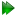Consistency testing script mode - the sample script

The following script can be found in the sample_scripts directory.

""" Script for consistency testing.

Severe artifacts can be introduced if model-free analysis is performed from inconsistent multiple magnetic field datasets. The use of simple tests as validation tools for the consistency assessment can help avoid such problems in order to extract more reliable information from spin relaxation experiments. In particular, these tests are useful for detecting inconsistencies arising from R2 data. Since such inconsistencies can yield artificial Rex parameters within model-free analysis, these tests should be use routinely prior to any analysis such as model-free calculations.

This script will allow one to calculate values for the three consistency tests J(0), F_eta and F_R2. Once this is done, qualitative analysis can be performed by comparing values obtained at different magnetic fields. Correlation plots and histograms are useful tools for such comparison, such as presented in Morin & Gagne (2009a) J. Biomol. NMR, 45: 361-372.

References
==========

The description of the consistency testing approach:

Morin & Gagne (2009a) Simple tests for the validation of multiple field spin relaxation data. J. Biomol. NMR, 45: 361-372. U{http://dx.doi.org/10.1007/s10858-009-9381-4}

The origins of the equations used in the approach:

J(0):
Farrow et al. (1995) Spectral density function mapping using 15N relaxation data exclusively. J. Biomol. NMR, 6: 153-162. U{http://dx.doi.org/10.1007/BF00211779}

F_eta:
Fushman et al. (1998) Direct measurement of 15N chemical shift anisotropy in solution. J. Am. Chem. Soc., 120: 10947-10952. U{http://dx.doi.org/10.1021/ja981686m}

F_R2:
Fushman et al. (1998) Direct measurement of 15N chemical shift anisotropy in solution. J. Am. Chem. Soc., 120: 10947-10952. U{http://dx.doi.org/10.1021/ja981686m}

A study where consistency tests were used:

Morin & Gagne (2009) NMR dynamics of PSE-4 beta-lactamase: An interplay of ps-ns order and us-ms motions in the active site. Biophys. J., 96: 4681-4691. U{http://dx.doi.org/10.1016/j.bpj.2009.02.068}
"""

# Create the data pipe.
name = 'consistency'
pipe.create(name, 'ct')

# Set up the 15N spins.
spin.name(name='N')
spin.element(element='N')
spin.isotope(isotope='15N', spin_id='@N')

relax_data.read(ri_id='R1_600',  ri_type='R1',  frq=600.0*1e6, file='r1.600.out', res_num_col=1, data_col=3, error_col=4)
relax_data.read(ri_id='R2_600',  ri_type='R2',  frq=600.0*1e6, file='r2.600.out', res_num_col=1, data_col=3, error_col=4)
relax_data.read(ri_id='NOE_600', ri_type='NOE', frq=600.0*1e6, file='noe.600.out', res_num_col=1, data_col=3, error_col=4)

# Generate the 1H spins for the magnetic dipole-dipole interaction.
sequence.attach_protons()

# Define the magnetic dipole-dipole relaxation interaction.
interatom.define(spin_id1='@N', spin_id2='@H', direct_bond=True)
interatom.set_dist(spin_id1='@N', spin_id2='@H', ave_dist=1.02 * 1e-10)

# Define the chemical shift relaxation interaction.
value.set(val=-172 * 1e-6, param='csa')

# Set the angle between the 15N-1H vector and the principal axis of the 15N chemical shift tensor
value.set(val=15.7, param='orientation')

# Set the approximate correlation time.
value.set(val=13 * 1e-9, param='tc')

# Set the frequency.
consistency_tests.set_frq(frq=600.0 * 1e6)

# Consistency tests.
minimise.calculate()

# Monte Carlo simulations.
monte_carlo.setup(number=500)
monte_carlo.create_data()
minimise.calculate()
monte_carlo.error_analysis()

# Create grace files.
grace.write(y_data_type='j0', file='j0.agr', force=True)
grace.write(y_data_type='f_eta', file='f_eta.agr', force=True)
grace.write(y_data_type='f_r2', file='f_r2.agr', force=True)

# View the grace files.
grace.view(file='j0.agr')
grace.view(file='f_eta.agr')
grace.view(file='f_r2.agr')

# Finish.
results.write(file='results', force=True)
state.save('save', force=True)

This is similar in spirit to the reduced spectral density mapping sample script (Chapter 8 on page).

The relax user manual (PDF), created 2020-08-26.